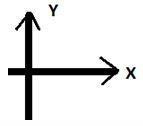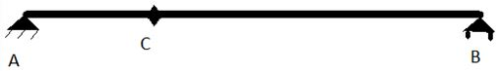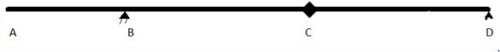Courses

# Test: Influence Lines For Beams

## 10 Questions MCQ Test Structural Analysis | Test: Influence Lines For Beams

Description
This mock test of Test: Influence Lines For Beams for Civil Engineering (CE) helps you for every Civil Engineering (CE) entrance exam. This contains 10 Multiple Choice Questions for Civil Engineering (CE) Test: Influence Lines For Beams (mcq) to study with solutions a complete question bank. The solved questions answers in this Test: Influence Lines For Beams quiz give you a good mix of easy questions and tough questions. Civil Engineering (CE) students definitely take this Test: Influence Lines For Beams exercise for a better result in the exam. You can find other Test: Influence Lines For Beams extra questions, long questions & short questions for Civil Engineering (CE) on EduRev as well by searching above.
QUESTION: 1

### While writing influence line equations, left most point is always considered as origin and following sign convention is followed.Q. If on ILD analysis peak force comes out to be 2 KN, then what will be the peak force if loading is 2KN?

Solution:

Explanation: Peak force will be load multiplied by earlier peak load i.e. 2*2.

QUESTION: 2

### Following figure is for Q2-Q7. AC= 1m, CB =3 m C is just an arbitrary point. A is pin support and B is a roller type support.Q. What will be the equation of ILD of shear force at point C for CB part?

Solution:

Explanation: Just assume force at any point between BC and conserve moment about point B.

QUESTION: 3

### Following figure is for Q2-Q7. AC= 1m, CB =3 m C is just an arbitrary point. A is pin support and B is a roller type support.Q. What will be the equation of ILD of shear force at point C for AC part?

Solution:

Explanation: Just assume force at any point between AC and conserve moment about point A.

QUESTION: 4

Following figure is for Q2-Q7.
AC= 1m, CB =3 m
C is just an arbitrary point. A is pin support and B is a roller type support.Q. If we have to apply a concentrated load in the above shown beam, such that shear at C becomes max. , where should we apply that load?

Solution:

Explanation: If we draw ILD according to the above given equations, we will see that peak of ILD comes at point C

QUESTION: 5

Following figure is for Q2-Q7.
AC= 1m, CB =3 m
C is just an arbitrary point. A is pin support and B is a roller type support.Q. If a concentrated load of 50KN is applied at point C, then what will be the shear developed at point C?

Solution:

Explanation: Position of ILD at point C is 0.75 (peak). So, shear developed will be 0.75 multiplied by 50KN.

QUESTION: 6

Following figure is for Q2-Q7.
AC= 1m, CB =3 m
C is just an arbitrary point. A is pin support and B is a roller type support.Q. What will be the shear developed at point C if a uniform load of 10KN/m is applied between point B and C?

Solution:

Explanation: In case of uniform load, area of ILD curve multiplied by uniform load gives the shear.

QUESTION: 7

Following figure is for Q2-Q7.
AC= 1m, CB =3 m
C is just an arbitrary point. A is pin support and B is a roller type support.Q. If both, a load of 50KN at point C and a uniform load of 10KN/m between CB acts, then what will be the shear generated at point C?

Solution:

Explanation: Net shear generated will be the sum of individually generated shear which has been already calculated earlier.

QUESTION: 8

Following figure is for Q8-Q10.
AB= 2m, BC= 3m, CD= 3m
B is pin support, D is roller and C is just an arbitrary point.Q. What will be the ILD equation for ILD of shear at point B?

Solution:

Explanation: Apply unit load at any point at a distance X and conserve moment about point D

QUESTION: 9

Following figure is for Q8-Q10.
AB= 2m, BC= 3m, CD= 3m
B is pin support, D is roller and C is just an arbitrary point.Q. What will be the ILD equation for ILD of shear at point C for AB part of beam?

Solution:

Explanation: Apply unit load between point A and B and conserve moment about point B.

QUESTION: 10

Following figure is for Q8-Q10.
AB= 2m, BC= 3m, CD= 3m
B is pin support, D is roller and C is just an arbitrary point.Q. What will be the ILD equation for ILD of shear at point D?

Solution: